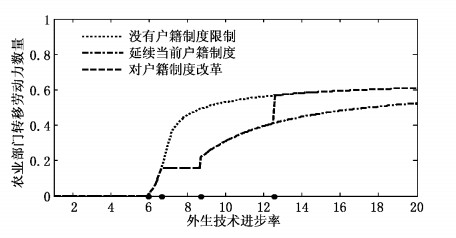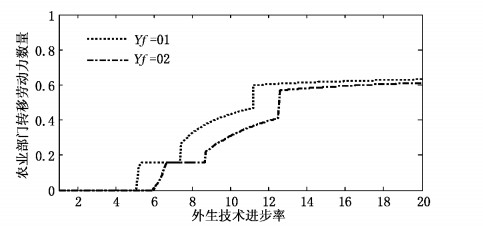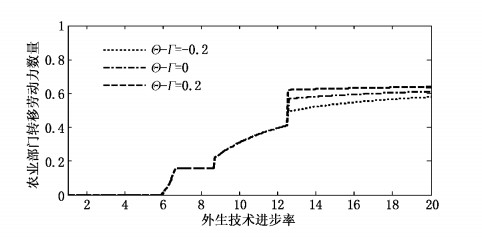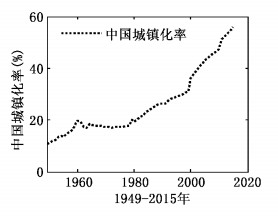﻿ 户籍制度改革对农村劳动力流动影响模拟研究——基于新经济地理学视角
 财经研究2016, Vol. 42Issue (10): 80-930

#### 文章信息财经研究2016年42卷第10期

Liu Junhui, Zhang Gu.

A Simulation Research of the Effect of the Reform of the Household Registration System on the Flow of Rural Labor Force: A Perspective of New Economic Geography

Journal of Finance and Economics, 2016, 42(10): 80-93.

### 文章历史《财经研究》
2016第42卷第10期

1.山西财经大学 经济学院，山西 太原 030006;
2.兰州大学 经济学院，甘肃 兰州 730000

A Simulation Research of the Effect of the Reform of the Household Registration System on the Flow of Rural Labor Force: A Perspective of New Economic Geography
Liu Junhui1, Zhang Gu2
1.School of Economics, Shanxi University of Finance and Economics, Taiyuan 030006, China;
2.School of Economics, Lanzhou University, Lanzhou 730000, China
Abstract: Based on the intermediate inputs model in new economic geography, this paper incorporates factors such as the household registration system, land property right system and the modes of the flow of labor force, and constructs the evolution model of the household registration system in China. Then it simulates the influences of the reform of the household registration system and land property right system on the flow of rural labor force by numerical calculation. It arrives at the conclusions as follows: firstly, different routes to the reform of the household registration system greatly affect the flow of rural labor force and economic development; if the restriction of the household registration system on the flow of labor force reduces, the economic system will release huge reform dividends, and if current household registration system remains, we will suffer great losses; secondly, different routes to the reform of the land property right system affect the results of the reform of the household registration system, and if the flow of rural labor force is accompanied by land, the dividends from the reform of the household registration system will enlarge. It shows that the reform of the household registration system and land property right system should be carried out together; not only the restriction of the household registration system on the flow of labor force should reduce, but also agricultural land contract rights and the right to the use of residence base should remain. It enriches & perfects current labor migration theory, and also provides a new idea for related decision-making of local governments.
Key words: reform of the household registration system    the flow of rural labor force    new economic geography    citizenization of migrant workers

(一) 基本假设

1.假设有两个地区R1R2。起初R2为核心地区，R1为边缘地区，即所有现代产业都位于地区R2，此外地区R1存在土地产权制度和户籍制度约束，地区R2没有。

2.假设经济系统农业部门A和现代产业部门M。其中农业部门规模收益递减，使用土地和劳动进行生产。现代部门规模收益递增，生产差异化产品，生产中需要投入可变投入和固定投入，二者均为劳动力和中间投入品组合的Cobb-Douglas函数，其中劳动所占份额为α，中间投入品组合所占份额为1-α，且中间投入品组合为CES型。

3.假定只有劳动力一种流动要素，每个地区劳动力是同质的，且劳动力数量为Ωrr=1, 2。劳动力不能在地区间流动，但是能在同一地区不同部门间流动。地区2劳动力在部门间完全自由流动，而地区1劳动力在不同部门之间流动情况见下面各阶段对应模型。

4.假定外生的技术进步率L，技术进步率主要影响劳动力的生产效率，而对土地数量没有影响。另外，假设每个地区的技术效率跟本国工业化程度有关，工业化程度越高，技术效率水平也越高。我们采用S型增长曲线对之进行修正，每个地区的技术进步率Lr=L×θ/(1+e-a(xr-b)+c)，abc为参数，x为本地区非农产业就业人口与农业就业人口之比，θ=(1+e-a(0-b)+c)，那么，各地区有效劳动力数量为Lr×Ωrr=1, 2。

5.为了简化，假设农产品没有运输成本，而工业产品为冰山型运输成本，并且工业品在本地区内部运输没有成本。

(二) 基本模型

1.消费者行为。所有消费者具有相同的偏好，具有相同的效用函数，上层效用函数为柯布-道格拉斯型，下层效用函数为CES型：

 $U=C_{A}^{(1-u)}{{({{C}_{M}})}^{u}}, {{C}_{M}}={{(\int{_{_{0}}^{n}}c{{(j)}^{\rho }}dj)}^{\frac{1}{\rho }}}, 0<\rho <1$ (1)

 ${{P}_{A}}{{C}_{A}}+G{{C}_{M}}=Y, G={{[\int _{0}^{n}p{{(j)}^{1-\sigma }}dj]}^{\frac{1}{1-\sigma }}}$ (2)

 $c(j)=uYp{{(j)}^{-\sigma }}/{{(G)}^{1-\sigma }}[\sigma \text{=}\frac{1}{(1-\rho )}\text{ }\!\!]\!\!\text{ }$ (3)

2.生产者行为。

(1)农业部门。当前土地产权制度赋予农民两种权利，农用地承包权和宅基地使用权。根据假设，农业部门生产函数A[(Ωr-λr)Lr]=K/η×[(Ωr-λr)Lr/K]η，ΩrλrLrK分别为r地区的劳动总数量、现代部门工人数量、技术进步率和土地要素投入量，η为参数。技术进步率Lr只改变有效劳动数量，而对土地数量没有影响，它等价于放大了地区r劳动力数量Lr倍。由农业部门生产函数对农业劳动力数量Ωr-λr求导，得到边际产出为：

 $\text{MP=}{{\text{A}}^{'}}\text{ }\!\![\!\!\text{ (}{{\Omega }_{r}}\text{-}{{\lambda }_{r}}\text{)}{{\text{L}}_{r}}\text{ }\!\!]\!\!\text{ }={{[({{\Omega }_{r}}-{{\lambda }_{r}})/K]}^{\eta -1}}\times \text{L}_{r}^{\eta }$ (4)

 $AP-A[\text{(}{{\Omega }_{r}}\text{-}{{\lambda }_{r}}\text{)}{{\text{L}}_{r}}]/\text{(}{{\Omega }_{r}}\text{-}{{\lambda }_{r}}\text{)}=(\frac{1}{\eta })\times {{[({{\Omega }_{r}}-{{\lambda }_{r}})/K]}^{\eta -1}}\times \text{L}_{r}^{\eta }$ (5)

 ${{w}_{1}}=\overline{{{w}_{A}}}-R\times ({{\lambda }_{r}}+{{\Omega }_{A}}-{{\Omega }_{r}})/{{\Omega }_{A}}\times {{p}_{A}};{{w}_{R}}=R\times \text{(}{{\Omega }_{r}}\text{-}{{\lambda }_{r}}\text{)}/{{\Omega }_{A}}\times {{p}_{A}}$ (6)

(2)现代部门。由前面假设，现代部门企业成本函数C(x)=PP(F+amx)，其中am为单位产出需要的可变投入组合量，x为产出水平，PP为劳动和投入品组合的价格指数。由于劳动和中间投入品组合形式为柯布-道格拉斯形式，所以要素组合的价格PP=wα(G)1-α，其中wG分别为工人的劳动工资水平和工业产品组合的价格指数。

 $p={{P}_{P}}{{\alpha }_{m}}(1-\frac{1}{\sigma });{{q}^{*}}=F(\sigma -1)/{{\alpha }_{m}}$ (7)

3.短期均衡。当市场达到短期均衡时，市场能够实现出清，此时产品供给等于需求。由工业品价格指数表达式可知，各地区工业品价格指数为：

 ${{G}_{r}}={{[\sum\limits_{s=1}^{2}{{{n}_{s}}}{{({{p}_{s}}{{T}_{sr}})}^{1-\sigma }}]}^{\frac{1}{1-\sigma }}}$ (8)

 ${{p}_{r}}=w_{r}^{\alpha }G_{r}^{1-\alpha }$ (9)

 ${{q}_{r, s}}={{({{p}_{r}})}^{-\sigma }}{{({{T}_{r, s}}/{{G}_{s}})}^{(1-\sigma )}}{{E}_{s}}$ (10)

 ${{q}_{r}}\equiv \sum\limits_{s=1}^{2}{{{q}_{r,s}}}={{({{p}_{r}})}^{-\sigma }}{{({{T}_{r,s}}/{{G}_{r}})}^{(1-\sigma )}}{{E}_{r}}+{{({{p}_{r}})}^{-\sigma }}{{({{T}_{r,s}}/{{G}_{s}})}^{(1-\sigma )}}{{E}_{s}}=q*$ (11)

 ${{E}_{r}}=u[{{Y}_{r}}-{{\Omega }_{r}}\overline{Y}]+(1-\alpha ){{n}_{r}}{{p}_{r}}{{q}_{r}}$ (12)

 ${{L}_{r}}{{\lambda }_{r}}{{w}_{r}}=\alpha {{n}_{r}}{{p}_{r}}{{q}_{r}}={{n}_{r}}{{p}_{r}}$ (13)

 ${{Y}_{r}}={{L}_{r}}{{\lambda }_{r}}{{w}_{r}}+A[\text{(}{{\Omega }_{r}}\text{-}{{\lambda }_{r}}\text{)}{{\text{L}}_{r}}]$ (14)

 ${{({{G}_{r}})}^{1-\sigma }}={{L}_{1}}{{\lambda }_{1}}{{({{T}_{1r}})}^{1-\sigma }}{{({{w}_{1}})}^{1-\sigma \alpha }}{{({{G}_{1}})}^{-\sigma (1-\alpha )}}+{{L}_{2}}{{\lambda }_{2}}{{({{T}_{2r}})}^{1-\sigma }}{{({{w}_{2}})}^{1-\sigma \alpha }}{{({{G}_{2}})}^{-\sigma (1-\alpha )}}$ (15)

 ${{[{{({{w}_{r}})}^{\alpha }}{{({{G}_{r}})}^{1-\alpha }}]}^{\sigma }}/\alpha ={{({{T}_{r1}}/{{G}_{1}})}^{1-\sigma }}{{E}_{1}}+{{({{T}_{r2}}/{{G}_{2}})}^{1-\sigma }}{{E}_{2}}$ (16)

 ${{Y}_{1}}={{L}_{1}}{{\lambda }_{1}}{{w}_{1}}+A[\text{(}{{\Omega }_{1}}\text{-}{{\lambda }_{1}}\text{)}\times {{\text{L}}_{1}}];{{Y}_{2}}={{L}_{2}}{{\lambda }_{2}}{{w}_{2}}+A[\text{(}{{\Omega }_{2}}\text{-}{{\lambda }_{2}}\text{)}\times {{\text{L}}_{2}}]$ (17)

 ${{E}_{1}}=u[{{Y}_{1}}-{{\Omega }_{1}}\overline{Y}]+{{L}_{1}}{{\lambda }_{1}}{{w}_{1}}(1-\alpha )/\alpha ;{{E}_{2}}=u[{{Y}_{2}}-{{\Omega }_{2}}\overline{Y}]+{{L}_{2}}{{\lambda }_{2}}{{w}_{2}}(1-\alpha )/\alpha$ (18)

 ${{L}_{r}}=L\times \theta /(1+{{e}^{-a({{x}_{r, -b}})}}+c), {{x}_{1}}/({{\Omega }_{1}}\text{-}{{\lambda }_{1}}), {{x}_{2}}={{\lambda }_{2}}/({{\Omega }_{2}}\text{-}{{\lambda }_{2}})$ (19)

(三) 我国户籍制度演变模型及长期均衡

1.第一阶段：1950年到1958年之前，人口自由流动。此时，假定地区1劳动力在农业部门和现代部门间自由流动，且不存在户籍歧视。当地区1现代部门潜在工资水平与农业部门工资水平相等时，地区1农业部门劳动力开始向现代部门转移，核心区产业也开始向地区1转移。我们设定产业转移活动一直持续到地区1产业份额和城市化率达到一定水平时，经济活动开始进入到第二阶段，此时地区1从农业部门向现代部门转移的劳动力数量为k1，此阶段两个地区的价格指数方程、工资方程、收入方程、支出方程及技术进步率方程如(15)式至(19)式。此时两个地区的农业部门效率单位工资水平分别为：

 $\overline{W_{A}^{1}}=\frac{A[({{\Omega }_{1}}\text{-}{{\lambda }_{1}})\times {{L}_{1}}]}{({{\Omega }_{1}}\text{-}{{\lambda }_{1}})\times {{L}_{1}}};\overline{W_{A}^{2}}=\frac{A[({{\Omega }_{2}}\text{-}{{\lambda }_{2}})\times {{L}_{2}}]}{({{\Omega }_{2}}\text{-}{{\lambda }_{2}})\times {{L}_{2}}}$ (20)

WA2=w2λ1=0，且WA1L1+Yfw1L1

w1L1WA1L1+Yf时，经济活动进入第二步，此时技术进步率临界值记为L1

WA2=w2w1L1=WA1L1+Yf，且λ1k1(λ1≠0)

λ1k1时，经济进入第二阶段，此时技术进步率记为L2

2.第二阶段：1958年到1978年，户籍制度严格限制农村人口向城市流动。这一阶段地区1农村劳动力不能再向现代部门流动。假设这期间外生技术进步率L增加量为t1，在这一阶段开始时，地区1已经有k1数量的农村劳动力转移到城市现代部门。那么只需将(15)式至(20)式中的λ1换成k1，其他变量都不需改变，在此不再赘述。

WA2=w2，且LL2+t1(t1为1958年起户籍制度开始实施到1978年改革开放时的技术进步率增量值)。这时地区1农业部门转移到城市现代部门的劳动力数量λ1即为k1

LL2+t1时，经济进入到第三阶段，此时外生技术进步率的临界值记为L3

3.第三阶段：从1978年到未来某一年开始进行户籍制度改革时。该阶段户籍制度对农民工的流动限制主要表现为“移民汇款”和“户籍歧视”，该部分需对二者进行处理。其中对“移民汇款”处理，假定农民工收入中有一部分用作最低生活保障，记为Y，剩余部分中有一个比例返回家乡，这个比例记为β，称为汇款系数，当β=1时，表示农民工非常节俭顾家，更接近老一代农民工的行为特征；当β=0时，自我意识比较强，与新一代农民工的行为更接近。对“户籍歧视”的处理有两种方式，一是假设农民工工资比城市劳动力工资少wf；二是假设农民工在基本迁移成本Yf之外还存在一个迁移成本wf，这两种处理方式的结果都是降低农民工净收入，本研究在建模过程中采用后者。当β=0，wf=0时，农民工跟城市居民完全一样，农村劳动力自由流动，这是户籍制度改革完成之后的理想状态。

WA2=w2w1×L1=[WA1×(Ω1-k1-Δλ1L1+βw1L1×Δλ1]/(Ω1-k1)+Yf+wf

LL3+t2时，经济进入到第四阶段，此时技术进步率的临界值记为L4，此阶段开始时，现代部门劳动力的就业数量k1+Δλ1值记为k2

4.第四个阶段：户籍改革完成时到其后任意一年。此时假设户籍制度改革的最终目标是人口的自由迁徙，此阶段模拟户籍制度改革完成后，人口流动处于完全自由流动状态时的情况。此时劳动力是永久性迁移，需根据城乡长期收入差距做出迁移决策。这时不再存在“户籍歧视”和“移民汇款”现象，当地区1农村劳动力搬到城市时存在一个搬迁成本Γf。这一阶段刚开始时，已经有k2单位的劳动力进入城市现代产业部门，将Δλ1记为该阶段从农村新转移劳动力数量。此时人口流动模式就又回到1950年到1958年状态。需将(15)式至(20)式中的λ1换成k2+Δλ1，其他变量都不需改变，在此不再赘述。

(1)租金留在农村WA2=w2V(0)=∫0+∞[w1(t)L1-wA1(t)]e-ρtdt+Θ-Γf-Yf=0

(2)租金带入城市WA2=w2V(0)=∫0+∞{[w1(t)L1+wR(t)]-wI(t)}e-ρtdt+Θ-Γf-Yf=0。图 1a 地区1农业部门劳动力转移情况 数据来源：中国统计年鉴。图 2 不同户籍制度改革路径的比较图 4 劳动力流动成本对农村劳动力转移的影响图 5 宅基地补偿金与城市迁入成本对农村劳动力转移的影响图 1b 新中国成立以来中国城镇化率 数据来源：中国统计年鉴。

(二) 不同土地产权制度改革路径对农村劳动力转移影响的比较图 3 土地产权制度改革对农村劳动力转移的影响

(三) 劳动力流动成本对农村劳动力转移的影响

(四) 宅基地补偿金与城市迁入成本对农村劳动力转移的影响

  安虎森, 颜银根, 朴银哲. 城市高房价和户籍制度:促进或抑制城乡收入差距扩大?——中国劳动力流动和收入差距扩大悖论的一个解释[J]. 世界经济文汇 , 2011 (4) : 41–54.  “城镇化进程中农村劳动力转移问题研究”课题组. 城镇化进程中农村劳动力转移:战略抉择和政策思路[J]. 中国农村经济 , 2011 (6) : 4–25.  邓曲恒, 古斯塔夫森. 中国的永久移民[J]. 经济研究 , 2007 (4) : 137–148.  都阳, 蔡昉, 屈小博, 等. 延续中国奇迹:从户籍制度改革中收获红利[J]. 经济研究 , 2014 (8) : 4–13.  胡枫, 史宇鹏. 农民工汇款与输出地经济发展——基于农民工汇款用途的影响因素分析[J]. 世界经济文汇 , 2013 (2) : 80–95.  梁琦, 陈强远, 王如玉. 户籍改革、劳动力流动与城市层级体系优化[J]. 中国社会科学 , 2013 (12) : 36–59.  陆铭. 玻璃幕墙下的劳动力流动——制度约束、社会互动与滞后的城市化[J]. 南方经济 , 2011 (6) : 23–37.  倪国华, 蔡昉. 农户究竟需要多大的农地经营规模?——农地经营规模决策图谱研究[J]. 经济研究 , 2015 (3) : 159–171.  倪鹏飞, 颜银根, 张安全. 城市化滞后之谜:基于国际贸易的解释[J]. 中国社会科学 , 2014 (7) : 107–124.  人口研究编辑部. 户籍制度50年[J]. 人口研究 , 2008 (1) : 43–50.  孙文凯, 白重恩, 谢沛初. 户籍制度改革对中国农村劳动力流动的影响[J]. 经济研究 , 2011 (1) : 28–41.  严超, 常志霄. 中国省际劳动力迁移对地区经济增长的影响研究——基于1995-2005年数据的实证分析[J]. 经济科学 , 2011 (6) : 19–29.  袁媛. 我国户籍制度改革中的路径依赖研究[J]. 农村经济 , 2015 (1) : 19–23.  张杰飞, 李国平, 柳思维. 中国农业剩余劳动力转移理论模型及政策分析:Harris-Todaro与新经济地理模型的综合[J]. 世界经济 , 2009 (3) : 82–95.  章莉, 李实, WilliamA, DarityJr. 中国劳动力市场上工资收入的户籍歧视[J]. 管理世界 , 2014 (11) : 35–46.  章莉, 李实. 中国劳动力市场就业机会的户籍歧视及其变化趋势[J]. 财经研究 , 2016 (1) : 4–16.  张伟进, 胡春田, 方振瑞. 农民工迁移、户籍制度改革与城乡居民生活差距[J]. 南开经济研究 , 2014 (2) : 30–53.  朱江丽, 李子联. 户籍改革、人口流动与地区差距——基于异质性人口跨期流动模型的分析[J]. 经济学(季刊) , 2016 (2) : 797–816.  Forslid R, Ottaviano G I P. An analytically solvable core-periphery model[J]. Journal of Economic Geography , 2003, 3 (3) : 229–240. DOI:10.1093/jeg/3.3.229  Fujita M, Krugman P, Venables A J. The spatial economics: Cities, regions, and international trade[M]. MA: MIT Press, 1999 .  Krugman P, Venables A J. The seamless world: A spatial model of international specialization[R]. NBER Working Paper No.5220, 1995.  Krugman P, Venables A J. Globalization and the inequality of nations[J]. Quarterly Journal of Economics , 1995, 110 (4) : 857–880. DOI:10.2307/2946642  Pflüger M, Südekum J. A synthesis of footloose-entrepreneur new economic geography models:When is agglomeration smooth and easily reversible?[J]. Journal of Economic Geography , 2008, 8 (1) : 39–54.  Pflüger M, Südekum J. Integration, agglomeration and welfare[J]. Journal of Urban Economics , 2008, 63 (2) : 544–566. DOI:10.1016/j.jue.2007.03.006  Russek S. Differential labour mobility and agglomeration[J]. Papers in Regional Science , 2010, 89 (3) : 587–606. DOI:10.1111/j.1435-5957.2009.00269.x  Tabuchi T, Thisse J F. Taste heterogeneity, labor mobility and economic geography[J]. Journal of Development Economics , 2001, 69 (1) : 155–177.  Todaro M P. A model of labor migration and urban unemployment in less developed countries[J]. American Economic Review , 1969, 59 (1) : 138–148.  Venables A J. Equilibrium locations of vertically linked industries[J]. International Economic Review , 1996, 37 (2) : 341–59. DOI:10.2307/2527327